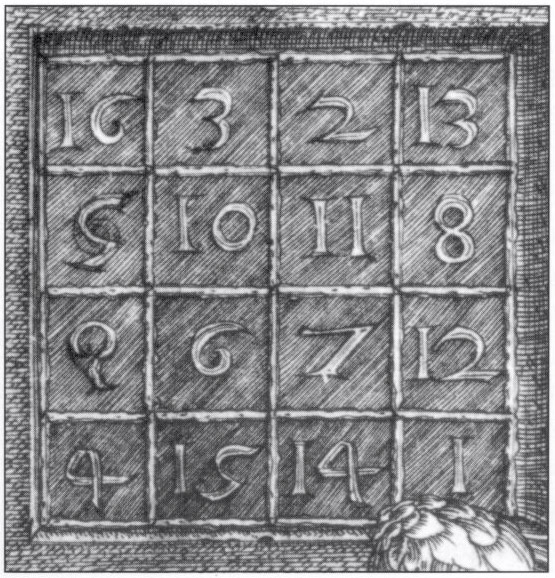# Dürer’s square

In Albrecht Dürer’s 1514 engraving Melancolia I, there is a famous 4×4 magic square. In addition to the rows, columns and main diagonals summing to 34, so do various other arrangements such as the quadrants and four corners.We can transform this into an equivalent magic square by decrementing each of the values. This will map the numbers into the interval [0, 15] instead of [1, 16]. We can then write these values in binary as four-bit numbers.

Of course, Dürer wouldn’t have done this as binary was unknown to Western civilisation at that time (Gottfried Leibniz was born more than a century later). You may begin to notice patterns in the binary square. These become much more evident when we separate it into ‘layers’.

Consider each layer separately. Each row, column, diagonal and any of a plethora of other arrangements (such as 2×2 ‘blocks’ in the corners) contains precisely two black and two white squares. This means that the sum must be equal to 2(1+2+4+8) = 30 in each of these arrangements. Since we decremented the values to obtain this square, the corresponding sum in Dürer’s original square is 34.

Remarkably, someone has found a magic cube containing Dürer’s square as a subplane. However, the numbers are not {1, 2, …, 64}, so it is of questionable validity. We can instead exploit the binary construction to yield a true magic cube with the numbers {1, 2, …, 64}. First, it is necessary to understand precisely what is happening in each of the binary bit planes.

We can construct the first bit plane by applying the exclusive or (XOR) operation to two ‘orthogonal’ patterns. If each of these orthogonal patterns contains two rows/columns (which they do!), then every row and column in the combined pattern must contain precisely two black and two white squares.

For the cube construction, we have to XOR together three orthogonal pairs of planes. We’ll choose planes which appear to generalise the two-dimensional counterparts, resulting in six ‘bitspaces’, two of which are shown below:

These two ‘bitspaces’ are linearly independent of each other and the other four bitspaces obtained by rotating them about a long diagonal. Hence, they together generate a magic cube related to Dürer’s square, where every orthogonal line has a sum of 130. Note that the four diagonals each pass through two cubes of each bitspace, so the diagonals (or, more generally, any centrally symmetric arrangement of four cubes) of the magic cube also have the same sum of 130. Additionally, selecting four out of the eight corner cubes (such that they form a regular tetrahedron) gives the sum of 130.

In fact, we can construct a 4x4x4x4 magic tesseract using this technique, and so on ad infinitum.

Amusingly, this visualisation of the tesseract is also a 16×16 magic square!

This entry was posted in Uncategorized. Bookmark the permalink.